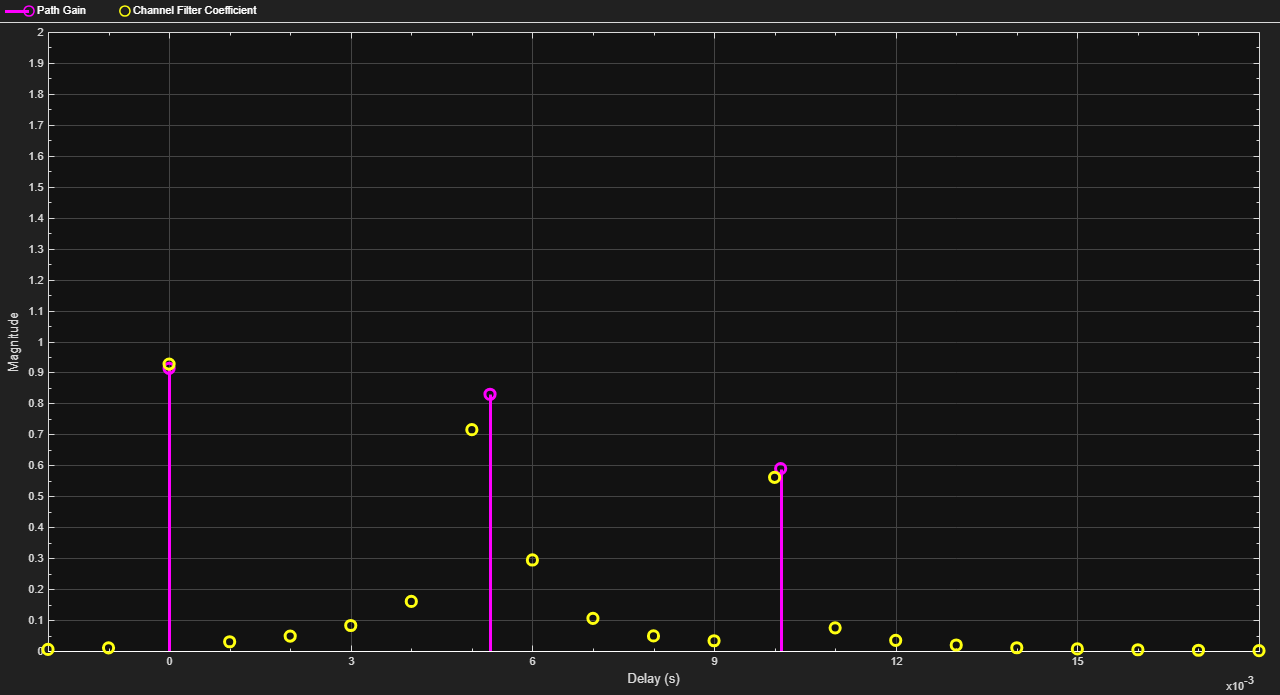Main Content

# channelDelay

Channel timing delay

## Syntax

``[delay,mag] = channelDelay(pathGains,pathFilters)``

## Description

example

````[delay,mag] = channelDelay(pathGains,pathFilters)` computes the channel timing delay by finding the peak of the channel impulse response. The function reconstructs the impulse response from a channel path gains array and a path filter impulse response matrix. The function returns the channel timing delay in samples, and the channel impulse response magnitude. For more information, see Channel Delay and Magnitude Computation.```

## Examples

collapse all

Configure a 2-by-2 MIMO channel. Use the `info` object function to retrieve the path filters.

```chan = comm.MIMOChannel('SampleRate',1000,'PathDelays',[0 1.5e-3], ... 'AveragePathGains',[1 0.8],'RandomStream','mt19937ar with seed', ... 'Seed',10,'PathGainsOutputPort',true); chanInfo = info(chan); pathFilters = chanInfo.ChannelFilterCoefficients;```

Compute the path gains by passing an impulse through the channel.

`[~,pathGains] = chan(ones(1,2));`

Compute the channel timing delay, specifying the retrieved path filters and computed path gains.

`delay = channelDelay(pathGains,pathFilters)`
```delay = 6 ```

Compute and show the relative timing delay for a Rayleigh channel over time.

Create a `comm.RayleighChannel` System object configured with three paths and impulse response visualization enabled.

```chan = comm.RayleighChannel; chan.SampleRate = 1e3; chan.PathDelays = [0 5.3e-3 10.1e-3]; chan.AveragePathGains = [0.1 1 0.5]; chan.PathGainsOutputPort = true; chan.RandomStream = 'mt19937ar with seed'; chan.Seed = 1; chan.Visualization = 'Impulse response'; chan.MaximumDopplerShift = 1;```

Use the `info` object function to retrieve the Rayleigh channel path filters. In a loop, pass a static signal of all ones through the Rayleigh channel. The `channelDelay` function uses the channel path gains array from each pass through the channel and the path filter coefficients, `chanInfo.ChannelFilterCoefficients` (returned by the `info` function) to compute the relative channel timing delay. The impulse response varies for each iteration. The impulse response for the last iteration is shown here. The `delay` vector shows the relative channel timing delay computed for each iteration.

```chanInfo = info(chan); numIter = 12; delay = zeros(1,numIter); for p=1:numIter [~,pg] = chan(ones(1e3,1)); delay(p) = channelDelay(pg,chanInfo.ChannelFilterCoefficients); end````delay`
```delay = 1×12 12 7 12 2 12 7 12 7 7 7 2 2 ```

## Input Arguments

collapse all

Channel path gains, specified as an Ncs-by-Np-by-Nt-by-Nr array, where:

• Ncs is the number of channel snapshots.

• Np is the number of paths.

• Nt is the number of transmit antennas.

• Nr is the number of receive antennas.

If any element in `pathGains` is `NaN`, the function assumes that no path exists between the transmitter and the receiver.

Data Types: `double` | `single`
Complex Number Support: Yes

Path filter impulse response, specified as an Np-by-Nh matrix. Np is the number of paths, and Nh is the number of impulse response samples.

Data Types: `double` | `single`
Complex Number Support: Yes

## Output Arguments

collapse all

Channel timing delay in samples, returned as an integer. This value represents the number of samples of delay relative to the first sample of the channel impulse response reconstructed from the `pathGains` and `pathFilters` inputs. The function computes the channel timing delay by finding the peak of the composite channel impulse response. For more information, see Channel Delay and Magnitude Computation.

Channel impulse response magnitude for each receive antenna, returned as an Nh-by-Nr matrix. Nh is the number of impulse response samples, and Nr is the number of receive antennas. For more information, see Channel Delay and Magnitude Computation.

## More About

collapse all

### Channel Delay and Magnitude Computation

The computation of the channel delay and impulse response magnitudes uses the composite channel impulse response.

The composite channel impulse response results from averaging the impulse response across all channel snapshots as represented in the path gains array. The input path gains array must be of the format Ncs-by-Np-by-Nt-by-Nr , where:

• Ncs is the number of channel snapshots.

• Np is the number of paths.

• Nt is the number of transmit antennas.

• Nr is the number of receive antennas.

The channel timing delay, output as a single value, is relative to the first sample of the channel impulse response. The function computes this value by finding the peak of the composite channel impulse response. The composite channel impulse response is the summation of the impulse responses across all transmit and receive antennas.

The receive impulse response magnitudes are output as an Nh-by-Nr matrix. Nh is the number of impulse response samples, and Nr is the number of receive antennas. To compute the receive impulse response magnitudes,

1. Path gains are summed across all channel snapshots.

2. The contribution from each path is added to the channel impulse response across all transmit and receive antennas.

3. The transmit antenna paths are combined in the channel impulse response array, leaving a matrix of impulse response samples versus receive antennas.

## See Also

Introduced in R2020a

## Support

#### Bridging Wireless Communications Design and Testing with MATLAB

Download white paper